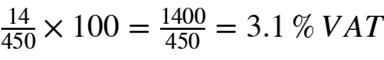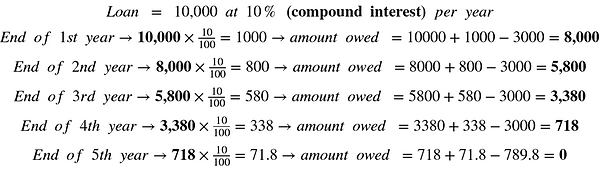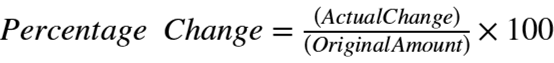###### Back to Mathematics

What does percentage mean? It is exactly as it sounds : per - cent - age where cent means 100 and centage means rate by the hundred. So percentage means per 100 parts.

Percentages are very useful because it can be standardized and used to compare data. For example all your grades in school are calculated in percentages because it is the standard. It is also easy to do calculations and comparisons with it. Which is why it is used in taxes, finance and banking, chemistry calculations, etc.

## Converting to Percentages and Back

Percentages are not that difficult to deal with as long as you know how to deal with fractions. Converting quantities into percentages is easy. All you have to do is first divide the original quantity into a 100 parts. Then you take take quantity that is being compared and see how many of the 100 parts it actually takes up.

Ratios and decimals are easily expressed as percentages by first converting them to fractions. Then you simply multiply that fraction by 100:If you cant remember how to convert decimals to fractions you can review by checking out thechapter.

Now that we know how to express numbers in percentages, how do we change percentages back to actual values. You just have to take the percentage and multiply it by the whole value. Let's look at a couple quick examples:As you can see it is pretty easy. You should have noticed that for percentages higher than 100 the calculated value is larger than the original quantity as shown above. So 100 % of a quantity = that quantity, whereas 200 % of that quantity = 2 times that quantity, 300% of that quantity = 3 times that quantity, etc. The last two calculation can also be calculated by breaking up the percentages :Let's do a couple word problems to understand how percentages are used simple interests and Value Added Tax (VAT).

Example 1

You want to buy a new smartphone because your current one is outdated. At the electronics store the price on the phone is \$450. However, when you are paying at the cashier, the bill says \$464 and you realize that this extra charge was the VAT. What percentage VAT did you have to pay?

So you had to pay \$14 (464 - 450 =14) extra because of tax. To calculate %VAT you simply take the \$14 as a fraction of the 450 and multiply by 100:A faster way to do this is to just take 464 as a fraction of 450:Since 100 % of the 103.1 % is the price of the phone without tax (\$450), the remaining 3.1% must be the VAT percentage.

Example 2

You deposit Rs.10,000 in your savings bank account at the start of the year. The simple interest rate at that bank is 6% a year. What is your account worth at the end of the year?

Since we are calculating simple interest rather than compound interest this question is easy to solve. Simple interest and compound interest are discussed in detail below. You just take 6% of 10,000  and then add that to 10,000 to get your end of year bank bank balance.There is another way to calculate this. You know that your Rs. 10,000 will increase due the 6% interest. This means you will still have the Rs.10000 which is a 100% plus the 6% increase. Therefore:Example 3

This time you deposit Rs. 10,000 in a checking bank account. This means there is no interest generated. However, assume you are charged 6% every year in bank charges. Pretty weird bank. What is your bank balance at the end of the year?

This is basically the opposite of Example 2. You take 6% of 10000 and substract it from 10000.Let's use the other method. This time you are losing money which mean you won't have 100% of your money at the end of the year. Instead there is a decrease of 6%. Therefore, 100% - 6% :## Interest and Percentage Change

Learning about simple interest and compound interest can be beneficial to you when you start making money as an employee or as an entrepreneur. These two types of interests may not seem too different when we are talking about money in terms of 2 or 3 years. However, if we are talking about money in terms of more than 5 years then these two types of interests produce very different results.

Simple Interest

Example 2 deals with simple interest. Simple interest is the interest calculated only on the principle amount invested or borrowed. So if we borrow \$10,000 at 10 %. Then the interest for every year is a 10% of \$10,000.As you can see every year you only get \$1,000 as interest. Now let's assume that your business takes off and you make an extra \$3,000 in profit. You want use this to pay off your loan and interest. Let's look at how it affects your simple interest:In this case you are using your profit to  pay of the debt. In the first year you get \$1000 in interest. But since you are paying \$3,000 that \$1,000 is cut off. The rest of \$2,000 is used to cut off the principal amount (\$10,000) you originally borrowed. This means your new loan amount is only \$8,000 and the simple interest is calculated on that now. At the end of the 5th year you only owe \$789.8 so you don't have to use up the whole \$3,000.

You should notice by now that paying off only your interest cannot save you money in simple interest because it is always calculated on the principal loan amount. However, if you pay off bits of the principal loan amount can save you money because the interest charges decreases as shown above.

Let's look at how compound interest is different:

Compound Interest

Compound interest is interest calculated on both the principal amount and all the interest that has not being paid off yet. Let's look at our previous example but this time use compound interest. \$10,000 at 10% compound interest every year. Then the interest for every year is 10% of \$10,000+interest accrued:The amount of interest owed increases every year. Whereas for simple interest it was fixed at \$1000. In fact the amount you owe at the end of the 5th year for compounded interest (\$16,105.1) is greater than what you owe at the end of the 6th year for simple interest (\$16,000).

If this was a 10 year loan; at the end of the 10th year under simple interest you would only owe \$20,000 but under compound interest you would owe \$25,937. This is a significant difference. However, if you pay off the interest on time every year then it would not be added to the principal amount when calculating interest for the following year. This means the amount owed at the end of each year will be a fixed \$10,000.

Let's once again assume that your business takes off and you make an extra \$3,000 in profit. You want use to this to pay off both your loan and interest. Let's look at how it affects your compounded interest:As you can see this is the exact same as that of the simple interest problem. This proves that as long as you pay up your interest without letting it accumulate you don't have to pay high interest regardless of simple interest or compound interest. If you are smart with your finances you can easily reduce losses.

Percentage Increase and Decrease

Example 4

Your uncle are currently being paid \$50,000 a year. He gets a promotion and his salary increases to \$65,000 a year. What percentage increase is this?

This is very easy to solve. They want to by how much your salary increase but in percentage. First you just have figure by how many dollars it increases: 65,000 - 50,000 = \$15000. Now you just have to convert it to a percentage. But do you take it as a fraction of the 65,000 or 50,000 to convert it to percentage? You have to take it as a fraction of 50,000 because you are calculating an increase.But if the current pay is \$65,000 and it decreases to \$50,000 then you need to calculate the percentage decrease. In this case you take 15,000 as a fraction of 65,000. This is shown below:By calculating percentage increase and decrease you are calculating a percentage change. The genral formual for this is:You can also modify the equation above slightly to use it to find percentage profit or loss:Now we know how to calculate the percentage change of a value. But how do we calculate a quantity's new value after it has undergone a percentage change. Its simple. You first calculate the actual change and then add it to the original amount. Assume you have to increase 550 by 11%:

Example 5You can calculate this faster by manipulating the percentage. The value increases, which means the new value will more than 100% of the original amount:The same process is followed when decreasing by a percentage. Now assume you decrease it by 11%:Since this time it is a decrease we know that the new value would be lower than a 100% of the original amount:Sometimes a specific quantity can undergo consecutive percentage changes. Solving these are not straight forward as adding them together. Let's look at a modfied version of Example 5:

Example 6

Assume your allowance is \$ 550. Since you were a good kid your parents increased it by 11%. But then you do something stupid and your parents decrease your allowance by 11%. What is your new allowance?

You might make the mistake of thinking that since your allowance is being increased by 11% then decreased by 11 % your allowance is the same. This is wrong.

You have to realize that the first change happens to your original allowance, \$550. But the second change occurs to your new allowance after the increase. The steps are shown below:As you can see your final answer is actually smaller than your original allowance. Let's do it using the other method: